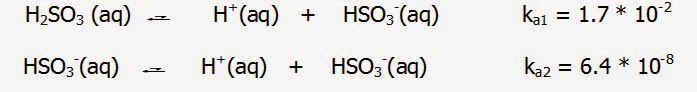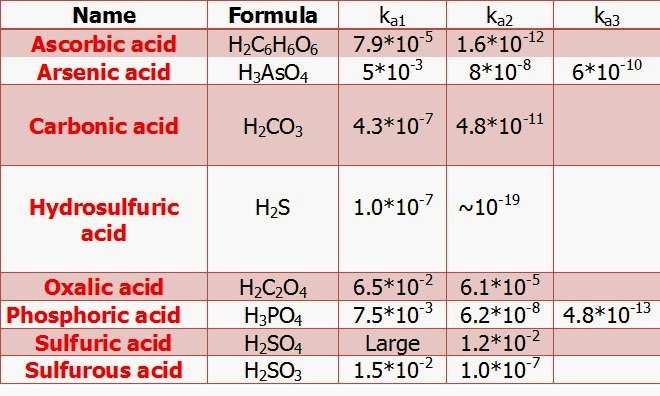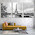Polyprotic Acids / pH Calculation | Chemistry Net

# Polyprotic Acids / pH Calculation

A large number of acids can give two or more protons on ionization (dissociation) and these are referred to as polyprotic acids. For example, with sulfurous acid (H2SO3) we have the successive ionizations:Fig. I.1: Stepwise dissociation of sulphurous acid (H2SO3)
A polyprotic acid always dissociates in a stepwise manner, one proton at a time. Note that the acid dissociation constants are labelled ka1 and ka2. The numbers on the constants refer to the particular proton of the acid that is ionizing. Thus, ka1 always refers to the equilibrium involving removal of the first proton of a polyprotic acid. Note also that ka2 for sulfurous acid is much smaller than ka1. This can be explained by the fact that the second H+ has to leave from a negatively charged species, HSO3- - electrostatic attraction has to be overcomed – while the first H+ from a neutral compound H2SO3.

The above observation is general: It is always easier to remove the first proton from a polyprotic acid than to remove the second and so on. The ka values become successively smaller as successive protons are removed.

The acid dissociation constants for common polyprotic acids are given in Table I.1Table I.1: Stepwise dissociation constants for several common polyprotic acids

#### Polyprotic Acids and Ionic Equilibria

Depending on the pH of the solution, a polyprotic acid may exist predominantly as the undissociated acid or any one of its anionic forms. It is easy to calculate the fraction present of the species involved in the equilibrium as a function of [H+].
As an example let us calculate the fraction of phosphoric acid present as a function of the pH. Phosphoric acid is typical of most weak polyprotic acids in that its successive ka values are very different.Fig. I.1: Stepwise dissociation of phosphoric acid
From the ionic equilibria shown above it is obvious that the relative acid strengths are:

H3PO4   >>  H2PO4-   >> HPO4-2

This means that in a solution prepared by dissolving H3PO4 in water, only the first dissociation step makes an important contribution to [H+]. This greatly simplifies the pH calculations for phosphoric acid solutions as is illustrated in the Example I.1 below.

Let us though first derive  general expressions for [H3PO4], [H2PO4-], [HPO4-2] as a function of [H+] in phosphoric acid solutions.

#### Polyprotic Acids – Phosphoric Acid - General Expression of the Equilibrium Concentrations (of the species involved as a function of  [H+])

Since there are six unknowns (the concentration of the acid, of the three conjugate bases and of H+ and OH-] we need six equations to define the relations between these quantities. These are

The equilibria ( equilibrium constant expressions):

[H+] * [H2PO4-] = ka1 * [H3PO4]     (1)

[H+] * [HPO4-2 ] = ka2 * [H2PO4-]  (2)

[H+] * [PO4-3] = ka3 * [HPO4-2]      (3)

[H+] * [OH-] = kw        (4)

The mass balance on phosphate:

[H3PO4] + [H2PO4-] +[HPO4-2] + [PO4-3] = C            (4a)

where C is the initial concentration of phosphoric acid.

The fraction of the acid present as each species is the ratio of the concentration of that species to the initial concentration C of the acid.

a3 = [H3PO4] / C

Note: The index on a shows the number of protons attached to the molecule. It is more convenient to calculate the reciprocal of this fraction, since this is directly expressible in terms of the mass balance (4a).

1/a3 = C/[H3PO4] = 1 + [H2PO4-] / [H3PO4] + [HPO4-2] / [H3PO4] + [PO4-3] / [H3PO4]   (5)

From equation (1):  [H2PO4-] / [H3PO4] = ka1 / [H+]                (6)

Multiplying (1) by (2) gives the third term of (5):

[HPO4-2] / [H3PO4] = (ka1* ka2)/ [H+]2    (7)

Multiplying (1) by (2) by (3) gives the fourth term of (5):

[PO4-3] / [H3PO4]    = (ka1* ka2* ka3)/ [H+]3    (8)

Substituting (6), (7) and (8) in (5) gives:

a3 = [H3PO4] / C = [ 1 + ka1 / [H+] + (ka1* ka2)/ [H+]2 + (ka1* ka2* ka3)/ [H+]3]-1     (9)

Combining (9) and (6):

a2 = [H2PO4-] / C = a3 * ka1 / [H+]    (10)

Combining (9) and (7):

a1 = [HPO4-2] / C = a3 * (ka1* ka2) / [H+]2    (11)

Combining (9) and (8):

ao = [PO4-3] / C = a3 * (ka1* ka2* ka3) / [H+]3     (12)

There are very few situations where the above exact relations are used. The above formulas should not be memorized since equilibrium concentrations for the species involved can be derived from equilibrium constant expressions and the assumption that all [H+] in solution is generated by the dissociation of H3PO4 (Example I.1).

Example I.1

Calculate the pH of a 5.0 M H3PO4 solution and determine equilibrium concentrations of the species involved

Solution

 DATA [H3PO4]initial = C = 5.0 M  ka1 = 7.5 * 10-3 ka2 = 6.2 * 10-8 ka3 = 4.8 * 10-13 UNKNOWNS [H3PO4] = ?  [H2PO4-] = ?  [HPO4-2] = ?  [PO4-3] = ?

Mathematical Approach (Exact Solution)

STEP 1: Write down the equilibrium reactions. Phosphoric acid H3PO4 is a weak triprotic acid – see the ka ‘s in Fig. I.1. It dissociates partially in water and most of the H+ is generated at the first dissociation reaction (notice that  ka1 >> ka2 >> ka3)

STEP 2: Write down the equilibrium constant expression and the equilibrium constant.

The dominant equilibrium will be the dissociation of H3PO4 and the equilibrium constant expression is given by:

ka1 = [H+] * [H2PO4-] / [H3PO4] = 7.5 * 10-3      (13)

STEP 3: Write down the equilibrium concentration of the species involved in the dominant equilibrium reaction.

Suppose that x M of H3PO4 dissociates in water

 H3PO4 H+ H2PO4- Initial 5.0 Μ 0 0 Change -x  M +x M +x M Final (at equilibrium) (5.0 – x) M x M x M

STEP 4: Substitute the equilibrium concentrations into the expression for ka1 and solve for x.

Since the value of x is small (5.0 – x) ~ 5

ka1 = [H+] * [H2PO4-] / [H3PO4] = (x)*(x) / (5.0 – x) ~ x2/5 =  7.5 * 10-3

and therefore x ~ 1.9*10-1 M,  [H+]= [H2PO4-] = x = 0.19 M,  pH = 0.72

The [H3PO4] = 5.0 – x = 4.8 M

The concentration of [HPO4-2] can be obtained from (11):

a1 = [HPO4-2] / C = a3 * (ka1* ka2) / [H+]2    (11)

The only unknown in the above equation is a3. It can be calculated from (9):

a3 = [H3PO4] / C = [ 1 + ka1 / [H+] + (ka1* ka2)/ [H+]2 + (ka1* ka2* ka3)/ [H+]3]-1 =
[ 1 + (7.5*10-3/ 0.19) + (7.5 * 10-3* 6.2 * 10-8)/(0.19)2 + (7.5 * 10-3* 6.2 * 10-8* 4.8 * 10-13)/(0.19)3]-1 = [ 1 + (0.039) + (1.288*10-8) + (3.258*10-20) ]-1 ~ 1 / 1.039 = 0.96

Therefore, a3 ~ 0.96    (12)

Substituting in (11) gives:

a1 = [HPO4-2] / 5 = a3 * (ka1* ka2) / [H+]2 = 0.96 * (7.5 * 10-3* 6.2 * 10-8) / (0.19)2 = 1.236 *10-8

Therefore, [HPO4-2] = 5 * 1.236 * 10-8 = 6.18 * 10-8 M  ~  6.2 * 10-8 M      (13)

Similarly, [PO4-3] can be calculated. [PO4-3] = 1.6 *10-19 M       (14)

“Chemical Approach” (Approximations)

Proceed as above up to the calculation of [H+] = [H2PO4-] = 0.19 M. The concentration of [HPO4-2] can be obtained from the equilibrium constant expression and ka2 (dissociation of H2PO4-).

H2PO4- (aq)    =   H+(aq)   + HPO4-2   (aq)       ka2 = 6.2 * 10-8       (15)

ka2 = [H+] * [HPO4-2 ] / [H2PO4-] = 6.2 * 10-8   (16)

Substituting the values for [H+] = [H2PO4-] in (16):

[HPO4-2 ] = ka2  = 6.2 * 10-8 M           (13)

Similarly, [PO4-3] can be calculated from the equilibrium constant expression and ka3 to give as above:

[PO4-3] = 1.6 *10-19 M                        (14)

The results show that the dissociation of H2PO4- and HPO4-2 is negligible relative to that of H3PO4. This is apparent from the fact that [HPO4-2] is 6.2 * 10-8 M – showing that only 6.2 * 10-8 mol/l of H2PO4- has dissociated. The value of [PO4-3] shows that the dissociation of HPO4-2 is even smaller.

For many polyprotic acids ka1 is much larger than subsequent dissociation constants, in which case the H+ in the solution comes almost entirely from the first dissociation reaction. As long as successive ka values differ by a factor of 103 or more, it is possible to obtain a satisfactory estimate of the pH of polyprotic acid solution by treating the acids as if they were monoprotic, considering only ka1.

In a manner analogous to a triprotic acid the following formulas can be derived for a diprotic acid of the general form H2A:

a2 =[H2A] / C = ([ 1 + ka1 / [H+] + (ka1* ka2)/ [H+]2)-1         (17)

a1= [HA-] / C = a2 * ka1 / [H+]            (18)

ao = [A-] / C = a2 * (ka1 * ka2)/ [H+]2                  (19)

Where ao , a1, a2 are the fraction of a diprotic acid present as each of the three species H2A, HA- and A-.
However, as for the triprotic acid there are very few situations where the above exact relations are used. The above formulas should not be memorized since equilibrium concentrations for the species involved can be derived from equilibrium constant expressions and the assumption that all [H+] in solution is generated by the dissociation of H2A.

For another solved example regarding pH calculation of a polyprotic acid please see the video below:

Relevant Posts

Solving Weak Acid and Weak Base pH problems

Weak Acid Weak Base pH calculation solved example

Weak Acids and Bases - Calculate the pH of a weak acid

Cubic Equation Calculator for Weak Acid-Base Equilibria

Self-ionization of water - Autoionization of water - The ion product of water (kw)

References

J-L. Burgot “Ionic Equilibria in Analytical Chemistry”, Springer Science & Business Media, 2012
J.N. Butler  “Ionic Equilibrium – Solubility and pH calculations”, Wiley – Interscience, 1998
Clayden, Greeves, Waren and Wothers “Organic Chemistry”, Oxford,
D. Harvey,  “Modern Analytical Chemistry”, McGraw-Hill Companies Inc., 2000
Toratane Munegumi, World J. of Chem. Education, 1.1, 12 (2013)
J.N. Spencer et al., “Chemistry structure and dynamics”, 5th Edition, John Wiley & Sons, Inc., 2012
L. Cardellini, Chem. Educ. Res. Pract. Eur., 1, 151, (2000)

Key Terms

polyprotic acids, dissociation, dissociation constants, phosphoric acid, concentration of the species as a function of [H+], conjugate base, equilibrium constant expressions, equilibrium concentrations, calculate the pH, monoprotic, ka,  triprotic acid, polyprotic acids and bases problems polyprotic acid and pka, polyprotic acid base equilibria, polyprotic acid concentration calculation, diprotic acid concentration calculation, polyprotic acids dissociation

#### 1 comment:

1.Sometimes it is inconvenient to use the concentration units. For example, a reactant’s concentration may change by many orders of magnitude. If we are interested in viewing the progress of the reaction graphically, ph calculator